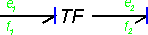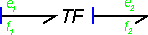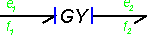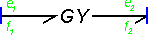﻿ 20-sim webhelp > Modeling Tutorial > Bond Graphs > Standard Elements > Transformers and Gyrators

# Transformers and Gyrators

Transformers and gyrators are bond graph elements that can convert energy ideally, as well in one physical domain as well as between one physical domain and another. A transformer is denoted by the mnemonic code TF and a gyrator by the code GY. In the table below the effort and flow equations are shown. The parameters r and n are the transformation ratio and gyration ratio. An examples of a transformer is a mechanical gear. An example of a gyrator is a DC-motor.

 TF-element bond graph element equation effort-in causalityf1 = (1/n) f2 e2 = (1/n) e1 effort-out causalityf2 = n f2 e1 = n e2 GY-element bond graph element equation effort-in causalityf1 = (1/r) e2 f2 = (1/r) e1 effort-in causalitye1 = r f2 e2 = r f1

The directions of the flow and effort are partly fixed for a transformer. Like the 1 junction and the 0 junction, the causality is constraint. For a transformer, an effort-in causality on the incoming bond results in an effort-out causality on the outgoing bond and vice-versa. For a gyrator, an effort-in causality on the incoming bond results in an effort-in causality on the outgoing bond and vice-versa.